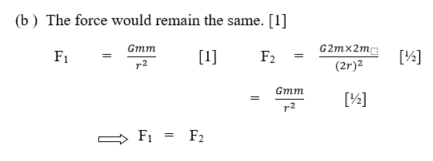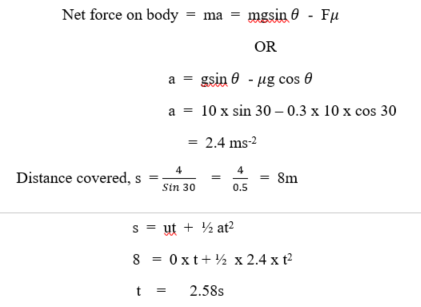Question 8

1. State two deductions that can be made from a displacement-time graph
2. If the distance between two equal masses is doubled and their individual masses are also doubled, what would happen to the force between them? Support your answer quantitatively
3. State two factors that affect the maximum height attained by a bullet fired from a gun.
1. State two practical examples of mechanical resonance.
2. A body is released from rest at the top of a plane inclined at 30o to the horizontal and 4.0 m high. If the coefficient of friction between the body and the plane is 0.3, calculate the time the body takes to reach the bottom of the plane.

Observation

Part (a) This was one of the popular choice of the candidates but the recorded performance was low. Candidates failed to mention two correct deductions from a displacement-time graph.

Part (b) Many candidates attempted this question but failed to score the full mark. The question basically tests the knowledge of Newton’s law of gravitation. Outcome showed that most candidates have difficulties with the concept. Performance was average.

Part (c) Performance was fair. Most candidates failed to see a projectile in the picture and failed to give two correct factors. Some candidates were able to state the two factors but failed to include “initial” in with the velocity.

Part (d) Majority of the candidates were unable to give correct examples of mechanical resonance. This implies that most candidates had a vague understanding of the principle of resonance. Some candidates listed instances of mechanical resonance like, a person on a diving board, matching soldiers on a bridge which is wrong. Performance was fair.
Performance was average.

Part (e) Majority of the candidates avoided this part. Less than half the number that attempted the question succeeded in calculating the length of the plane but failed to use the correct equation in calculating the time. Performance was low.

8. (a)    Displacement – time graph.
Any correct 2 x 1 mark
-      Uniform velocity
-       Instantaneous velocity
-       Initial displacement.(c)        Maximum height of a projectile
Any correct 2 x 1 mark             =
e.g  -  initial velocity / speed (u)
-  angle of projection ()
-  acceleration due to gravity (g)
-  air resistance

(d)         Mechanical Resonance
Any correct 2 x 1 mark
e.g – a diver attaining maximum uplift on a diving board
-  collapse of a bridge (by matching soldiers)
-   car bodies rattle (at very high speed)
-  shattering of glass (due to high pitch notes)
-   Throwing off a paper rider (from a vibrating stretched string)
(Accept any other valid example)
(e)         Let the body move down the inclined plane with acceleration = a